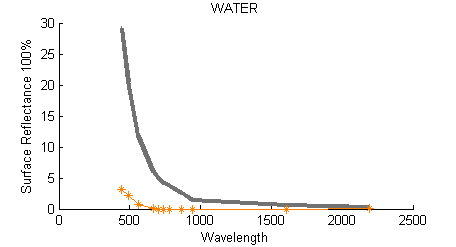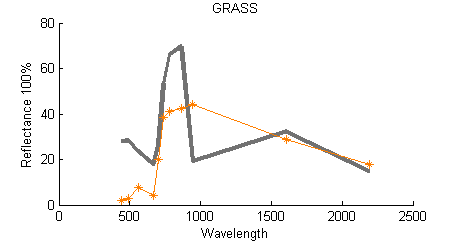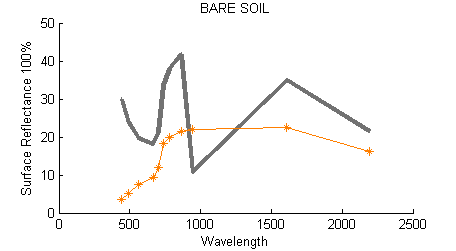# Atmospheric Correction (from TOA to BOA)Hi,

I used Sen2Cor software for atmospheric correction and it produces logical values for every single pixel.

The question is, how Sen2Cor can compute good BOA Reflectance using bad TOA Reflectance?

TOA Reflectance is computed as : TOA_Digital_Number / Scale_Factor, where scale factor=10,000 . Is it correct?

If it is correct, the most pixels in the scene have greater values than the reality, and in some bands the values are greater than 100%, even more than 200%. This is completely wrong. So how Sen2Cor computes good BOA using bad TOA?

I can think 2 ways which it can happen

1st TOA_Reflectance no equal with TOA_Digital_Number / 10,000

2nd L1B —> wrong computation ----> L1C ----> reverse wrong computation —> L2A

Am I wrong? Does anyone knows how to compute the correct TOA reflectance?

I really need it!

Reflectance is computed as you describe: TOA_Digital_Number / Scale_Factor, where scale factor should be 10.000 at present time. You can check the scale factor in the metadata.

Regards
bdpg

I used the image : S2A_OPER_PRD_MSIL1C_PDMC_20160109T201540_R007_V20160109T091904_20160109T091904

And it has similar values with all images that i used.
S2A_OPER_PRD_MSIL1C_PDMC_20160203T163603_R050_V20160201T091841_20160201T091841
S2A_OPER_PRD_MSIL1C_PDMC_20160216T022321_R007_V20160129T090458_20160129T090458
S2A_OPER_PRD_MSIL1C_PDMC_20160112T183456_R050_V20160112T092801_20160112T092801

As you can see here http://seom.esa.int/S2forScience2014/files/01_S2forScience-MethodsII_MULLER-WILM.pdf (page 19) : TOA Reflectance values aren’t more different than BOA Reflectance values. This is the normal.

At big wavelengths the scattering is not importand, when you have a clear day (low aod, 0.126 in my image) so the absorption makes the big different between TOA and BOA reflectance. That meens that BOA >= TOA at these wavelengths… This is correct as you can see in the precedent pdf. But it doesn’t agree with the next results :

(band 9)

BOA ~ TOA /2 This is imposible for a clear day, but the BOA values seems correct.
(grey = toa, orange = boa)TOA seems ~ 2 time bigger than normal.

So i can think two ways it can happen:

1. TOA=DN/10000, scale factor 10000 is wrong, the correct would be something like TOA=DN/20000 .
2. They computed wrong TOA Reflectance, maybe something like TOA_REF = 2*π * Lsat / Esun / cos(θ) , when the real formula is TOA_REF = π * Lsat / Esun / cos(θ), and the do the reverse mistake in Sen2Cor, so the one mistake negates the other, and it produce correct BOA.
1 Like

Hello!

Very interesting analysis, thank you for that!
Has there been a follow-up on the matter?